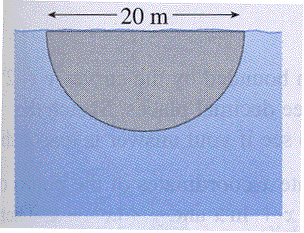A vertical plate is submerged in water and has the shape as seen in the picture. Using the facts that the density of water is $1000\; kg/m^3$ and acceleration due to gravity is $9.8\; m/s^2,$ calculate the hydrostatic force (in N) against the end of the tank. Make sure your answer is correct to the nearest thousand.

(Hint: First set up a Riemann sum that approximates the hydrostatic force, which can then be used to obtain an integral that represents the force.)Hydrostatic force = N